Search by Topic

Resources tagged with Quadrilaterals similar to Hexagon Transformations:

Filter by: Content type:
Age range:
Challenge level:

There are 33 results

Broad Topics > Angles, Polygons, and Geometrical Proof > Quadrilaterals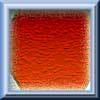Hexagon Transformations

Age 7 to 11 Challenge Level:

Can you cut a regular hexagon into two pieces to make a parallelogram? Try cutting it into three pieces to make a rhombus!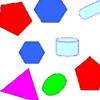Part the Polygons

Age 7 to 11 Short Challenge Level:

Draw three straight lines to separate these shapes into four groups - each group must contain one of each shape.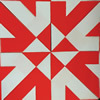Folding Flowers 1

Age 7 to 11 Challenge Level:

Can you visualise what shape this piece of paper will make when it is folded?Age 7 to 11 Challenge Level:

This practical problem challenges you to make quadrilaterals with a loop of string. You'll need some friends to help!Fred the Class Robot

Age 7 to 11 Challenge Level:

Billy's class had a robot called Fred who could draw with chalk held underneath him. What shapes did the pupils make Fred draw?Age 11 to 14 Challenge Level:

Four rods, two of length a and two of length b, are linked to form a kite. The linkage is moveable so that the angles change. What is the maximum area of the kite?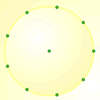Age 7 to 11 Challenge Level:

How many DIFFERENT quadrilaterals can be made by joining the dots on the 8-point circle?Poly Plug Rectangles

Age 5 to 14 Challenge Level:

The computer has made a rectangle and will tell you the number of spots it uses in total. Can you find out where the rectangle is?Cut and Make

Age 7 to 11 Challenge Level:

Cut a square of paper into three pieces as shown. Now,can you use the 3 pieces to make a large triangle, a parallelogram and the square again?Opposite Vertices

Age 11 to 14 Challenge Level:

Can you recreate squares and rhombuses if you are only given a side or a diagonal?Folding

Age 7 to 11 Challenge Level:

What shapes can you make by folding an A4 piece of paper?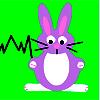Rabbit Run

Age 7 to 11 Challenge Level:

Ahmed has some wooden planks to use for three sides of a rabbit run against the shed. What quadrilaterals would he be able to make with the planks of different lengths?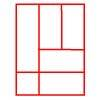Is a Square a Rectangle?

Age 7 to 11 Challenge Level:

How many rectangles can you find in this shape? Which ones are differently sized and which are 'similar'?Age 11 to 16

This gives a short summary of the properties and theorems of cyclic quadrilaterals and links to some practical examples to be found elsewhere on the site.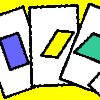Age 11 to 14 Challenge Level:

A game for 2 or more people, based on the traditional card game Rummy. Players aim to make two `tricks', where each trick has to consist of a picture of a shape, a name that describes that shape, and. . . .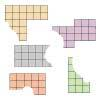Torn Shapes

Age 7 to 11 Challenge Level:

These rectangles have been torn. How many squares did each one have inside it before it was ripped?Age 11 to 14 Challenge Level:

We started drawing some quadrilaterals - can you complete them?Rectangle Tangle

Age 7 to 11 Challenge Level:

The large rectangle is divided into a series of smaller quadrilaterals and triangles. Can you untangle what fractional part is represented by each of the shapes?Triangle Transformation

Age 7 to 14 Challenge Level:

Start with a triangle. Can you cut it up to make a rectangle?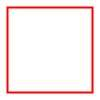Numerically Equal

Age 7 to 11 Challenge Level:

Can you draw a square in which the perimeter is numerically equal to the area?Shapely Pairs

Age 11 to 14 Challenge Level:

A game in which players take it in turns to turn up two cards. If they can draw a triangle which satisfies both properties they win the pair of cards. And a few challenging questions to follow...Property Chart

Age 11 to 14 Challenge Level:

A game in which players take it in turns to try to draw quadrilaterals (or triangles) with particular properties. Is it possible to fill the game grid?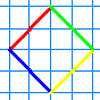A Cartesian Puzzle

Age 7 to 11 Challenge Level:

Find the missing coordinates which will form these eight quadrilaterals. These coordinates themselves will then form a shape with rotational and line symmetry.Bracelets

Age 7 to 11 Challenge Level:

Investigate the different shaped bracelets you could make from 18 different spherical beads. How do they compare if you use 24 beads?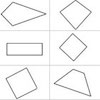Age 7 to 11 Challenge Level:

A task which depends on members of the group noticing the needs of others and responding.Transformations on a Pegboard

Age 7 to 11 Challenge Level:

How would you move the bands on the pegboard to alter these shapes?Making Rectangles, Making Squares

Age 11 to 14 Challenge Level:

How many differently shaped rectangles can you build using these equilateral and isosceles triangles? Can you make a square?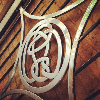Age 11 to 14 Challenge Level:

How many questions do you need to identify my quadrilateral?Cutting it Out

Age 5 to 11 Challenge Level:

I cut this square into two different shapes. What can you say about the relationship between them?Fractions Rectangle

Age 11 to 14 Challenge Level:

The large rectangle is divided into a series of smaller quadrilaterals and triangles. Can you untangle what fractional part is represented by each of the ten numbered shapes?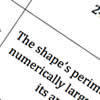Shape Draw

Age 7 to 11 Challenge Level:

Use the information on these cards to draw the shape that is being described.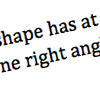What Am I?

Age 7 to 11 Challenge Level:

Can you draw the shape that is being described by these cards?An Equilateral Triangular Problem

Age 11 to 14 Challenge Level:

Take an equilateral triangle and cut it into smaller pieces. What can you do with them?